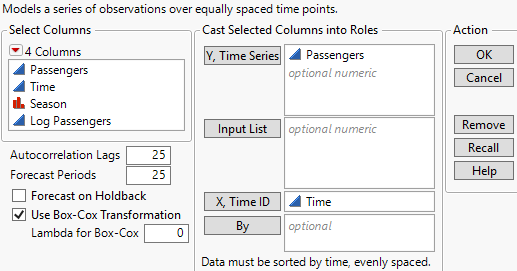Predictive and Specialized Modeling > Time Series Analysis > Launch the Time Series Platform
Publication date: 11/10/2021

# Launch the Time Series Platform

Launch the Time Series platform by selecting Analyze > Specialized Modeling > Time Series. The Time Series launch window for the Seriesg.jmp sample data table is shown in Figure 18.4.

Figure 18.4 The Time Series Launch WindowThe Time Series platform launch window contains the following options:

Y, Time Series

Assigns one or more columns as time series variables. Displayed on the Y axis.

Input List

Assigns one or more columns as input series variables. Displayed in the Input Time Series Panel and used in transfer function models. The input series variable must be numeric, either as a time series or an indicator.

X, Time ID

Assigns one variable for labeling the time axis (X axis). If no variable is specified for Time ID, the row number is used instead.

Note: If you use an X, Time ID variable, you can specify the time frequency by using the Time Frequency column property. You can choose Annual, Quarterly, Monthly, Weekly, Daily, Hourly, By Minute, and By Second. This helps JMP determine the spacing of the data when plotting the forecast values. If no frequency is specified, the data is treated as equally spaced numeric data.

Caution: It is assumed that the observations of the variable assigned to X, Time ID are equally spaced. However, the Time Series platform checks only whether the time stamps are increasing. The platform does not check if the observations are equally spaced.

By

Assigns a column that creates a report consisting of separate analyses for each level of the variable. If more than one By variable is assigned, a separate analysis is produced for each possible combination of the levels of the By variables.

Note: If you use a By variable, you might have to change the number of autocorrelation lags depending on how many observations there are for each level of the By variable. The number of lags must be greater than one but less than the number of observations per level.

Autocorrelation Lags

Specifies the number of lags to use in computing the autocorrelations and partial correlations. This is the maximum number of periods between points used in the computation of these correlations. It must be greater than one but less than the number of rows. The default number of lags is 25.

Tip: A commonly used rule for the maximum number of lags is n/4, where n is the number of observations.

Forecast Periods

Specifies the number of observations that are forecast using each model fitted to the data. The default number of forecasts is 25.

If you select the Forecast on Holdback option, the Forecast Periods value specifies the number of observations at the end of the series that are not included in the model fitting. These values are then forecast using the fitted model.

If you do not select the Forecast on Holdback option, the Forecast Periods value specifies the number of future observations that are forecast after the end of the series.

Forecast on Holdback

Determines whether forecasts are made on future observations or on the holdback observations. If the Forecast on Holdback option is selected, the forecasts are made on the holdback set that is determined by the number specified in the Forecast Periods option.

Use Box-Cox Transformation

Transforms the original data using a Box-Cox transformation with the lambda that is specified in the Lambda for Box-Cox option. When this option is selected, all analyses in the Time Series report are performed on the transformed data. However, all forecasts are transformed back and reported on the original scale.

Lambda for Box-Cox

(Available only when Use Box-Cox Transformation is selected.) Specifies the lambda parameter used for the Box-Cox transformation of the original data.

Note: In order to preserve the order and spacing of a time series, the Time Series platform treats excluded rows in the data table as missing values.